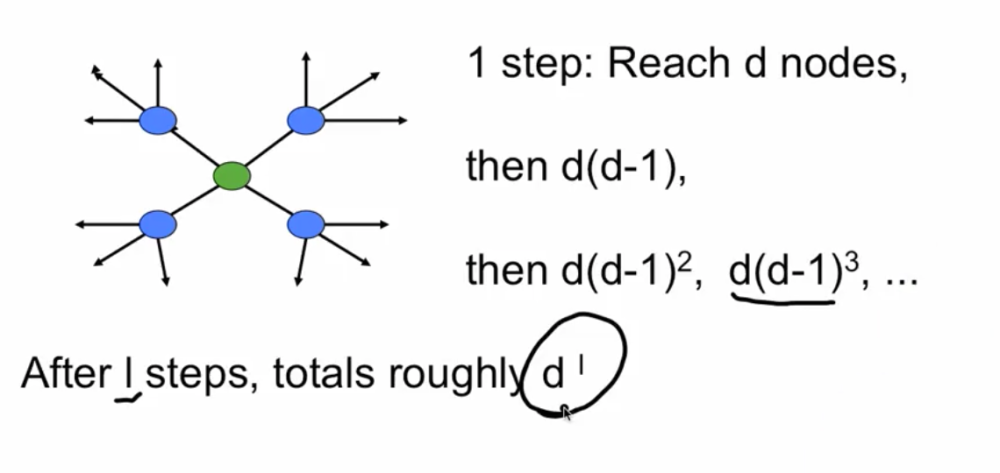# Diameter¶

• Bounds can be difficult - theorems are narrow, but intuition is easy

• Let's start with an easy calculation

• Cayley Tree: each node besides leaves has degree d

# Ideas¶• Moving out "l" links form root in each direction reaches $d + d(d-1) + ...d(d-1)^{l-1}$ nodes

• $d + d(d-1) + ...d(d-1)^{l-1}$

$= \frac{d((d-1)^l - 1)}{d-2}$

$\approx (d-1)^l$ for a reasonable larege d

• To reach n-1, the rest of all the nodes, need roughly $(d-1)^l = n$ or n-1 roughly

• or "l" on the order of $\frac{log(n)}{log(d)}$

• $\frac{log(n)}{log(d)}$ is exactly number about Erdos-Renyi random graph. If you just put out a random graph, we're getting something like that. So if we had a really regular tree, we would end up having this kind of calculation for how long it took to reach somebody at the center to reach the other ones. The overall diameter is going to be on the order of twice this, but roughly propotional to that.

What if not a tree, but E-R randm graph?

• all have same degree - really are random

• show that fraction of nodes that have nearly average degree is going to 1
• some links may double back

• most nodes until the last step are still not reached!